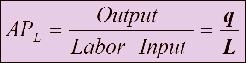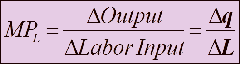Select Page

## Why do we need to know about Average and Marginal Product ?

When we are talking about Production with One variable or in other words when we a firm is producing something with the limited constraint of having a fixed variable , say Capital in the Short-Run. Also, only being able to change another variable say Labor and it is in a tough competition of increasing its Output. There comes the part where we need to clear the concept of Marginal and Average Product of that particular input we are changing (Labor)

## What is Average Product ?

Average Product is the Output per unit work by Input used (Labor)

It is calculated as :This formula basically tells us the productivity of the firms labor on an average. That is why we use the word PER-UNIT.

## What is Marginal Product ?

Marginal means Additional, So Marginal Product is the Additional product/output that is produced by one unit increase in labor

Its is calculated as :We use Marginal Product in cases when we want to know that either an additional labor (which we are considering as the flexible input) is producing more output or is it in fact decreasing the output levels on average. In other words, how much does an additional Labor(input) contributes to the production level which is what we call as the Marginal Product of labor.

In the Next topic we will discuss how Marginal and Average Product Curves explain the Production levels.

Shares